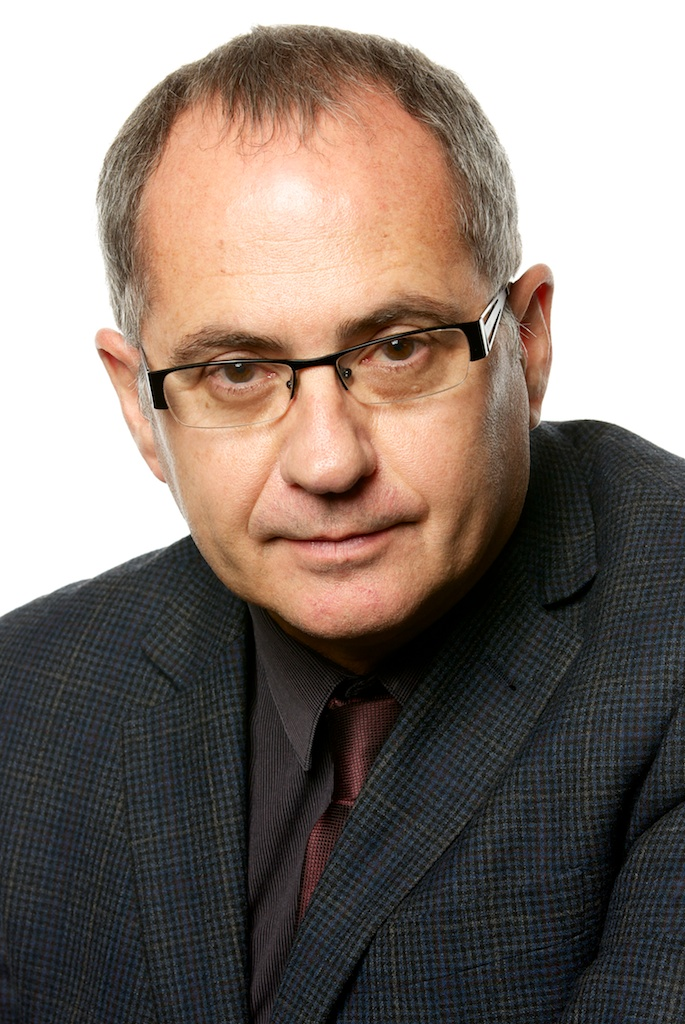Researcher

# Emeritus Professor Bruce Ian Henry## My Expertise

nonlinear dynamics; chaos; fractals; pattern formation

## Fields of Research (FoR)

Dynamical Systems in Applications, Stochastic Analysis and Modelling, Complex Physical Systems, Statistical Mechanics, Physical Combinatorics and Mathematical Aspects of Condensed Matter, Applied Mathematics not elsewhere classified

## Biography

### Biography

My research is in the broad area of nonlinear and statistical science with particular interests in; pattern formation, random walks, anomalous diffusion, fractional calculus, applications of chaos and fractals, mathematical modelling, stochastic modelling, neuronal degeneration, spread of infectious diseases, growth of stromatolites, nonlinear lattice dynamics and statistical mechanics. My current work is focussed on...view more

### Biography

My research is in the broad area of nonlinear and statistical science with particular interests in; pattern formation, random walks, anomalous diffusion, fractional calculus, applications of chaos and fractals, mathematical modelling, stochastic modelling, neuronal degeneration, spread of infectious diseases, growth of stromatolites, nonlinear lattice dynamics and statistical mechanics. My current work is focussed on mathematical models for subdiffusive transport.

My teaching is in applied mathematics areas of calculus, differential equations, dynamical systems.

### Education

• PhD "Nonlinear Vibrational Excitations in a Diatomic Chain", Supervised by Jaan Oitmaa (Theoretical Physics) and Alec Opie (Applied Mathematics), UNSW 1985
• BSc(Hons I), Theoretical Physics, UNSW 1981

### Training

• QEII Fellow, Institute for Advanced Studies, ANU, 1989-1991
• Postdoctoral Fellow, Institute for Advanced Studies, ANU, 1987-1989
• Postdoctoral Fellow, University of Waterloo, Canada, 1984-1987

## RESEARCH

### My Research Goals

• Develop mathematical methods and models to describe subdiffusive transport in complex environments
• Develop mathematical models for electrotonic properties of spiny neurons
• Develop mathematical models for spread of infectious diseases in complex systems

### My Research in Detail

My research is concerned with the development and application of mathematical methods from Nonlinear Dynamical Systems and from Statistical Physics to model, non-equilibrium behaviour, pattern formation, transport, and self-organization in complex systems. I have been publishing in this area for more than thirty years.

Over the past decade I have been working at the forefront of research on developing, analyzing and simulating mathematical models for sub-diffusion. Mathematical models of diffusion processes are widely used across a broad range of disciplines in systems involving random motions or random fluctuations. Famous models in this area include the Fokker-Planck equation, the Feynman-Kac equation and the Black-Scholes equation. One of the most fundamental results of standard diffusion is that the mean square displacement of the diffusing entities scales linearly with time. This result was the centrepiece of both Einstein's classic 1905 paper on Brownian motion and Bachelier's pioneering 1900 PhD thesis on pricing financial options, and it is consistent with the Central Limit Theorem of probability theory. Over the past few decades, sophisticated experiments and data collection in numerous systems have revealed experimental measurements of mean square displacements that scale slower than linearly with time. Diffusive transport with this property is called sub-diffusion. My major contribution has been the development of a new mathematical paradigm, fractional reaction diffusion equations, for modelling pattern formation and signalling in systems with sub-diffusion, reactions and forcing. My group introduced the first fractional reaction-sub-diffusion equation from the theory of continuous time random walks (Henry and Wearne, 2000). We carried out the first studies of Turing pattern formation in fractional reaction-diffusion systems (Henry and Wearne, 2002, Henry, Langlands and Wearne, 2005). We introduced the first implicit numerical scheme for solving fractional diffusion equations (Langlands and Henry, 2005). We generalized the continuous time random walk framework to include linear reaction kinetics (Henry, Langlands and Wearne, 2006). We extended subdiffusive transport models to include forcing from chemotaxis (Langlands and Henry, 2010). We derived the correct fractional Fokker-Planck equation to model subdiffusive trasnport with forcing from general space-and-time dependent forces (Henry, Langlands and Straka, 2010). We have also developed a fractional cable equation model to describe sub-diffusive transport in spiny dendrites of nerve cells (Henry, Langlands and Wearne, 2009, Langlands, Henry and Wearne, 2011). Recently we have begun to extend transport models with reactions and forcing to networks (Angstmann, Donnelly, Henry, 2013).

### Current Student Projects (PhD and Honours)

• Subdiffusive transport on networks
• Spread of infectious diseases on networks
• Stochastic resonance in systems with subdiffusive transport

Be open minded, be confident, follow the path suggested by your supervisor until you know enough not to. Talk to other students, socialize. Be prepared to work hard for long periods of time. Talk to your supervisor. Join the team. Learn how to develop magical powers.

### Recent Grants

• ARC Discovery Project 2014, 2015, 2016 "Fractional subdiffusion: Modelling and computation"
• ARC Discovery Project 2013, 2014, 2015 "Mathematical modelling can provide vital information on the effectiveness and practical implementation of microbicides and vaccines against HIV"
• ARC Discovery Project 2010, 2011, 2012 "New mathematics of fractional diffusion for understanding cognitive impairment at the neuronal level"
• ARC Discovery Project 2006, 2007, 2008 "Mathematical measurement and modelling of neuronal degeneration"

## TEACHING & OUTREACH

### Courses I Teach

#### Recent Years

• MATH2120 Differential Equations
• MATH2281 Biomathematics
• MATH3041 Mathematical Modelling for Real World Systems
• MATH3201 Dynamical Systems and Chaos
• MATH5215 Modern Topics in Dynamics

### Professional affilliations and service positions

• Head of School, School of Mathematics and Statistics
• Deputy Chair, Australian Council for Heads of Mathematical Sciences
• Editorial Board, Parabola
• Fellow, Australian Institute of Physics
• Fellow, Australian Mathematical Society
• Member, American Physical Society
View less

## Location

School of Mathematics and Statistics
UNSW Sydney
NSW 2052
The Red Centre
Room 3075

9385 7001
9385 7123

## Publications

by Emeritus Professor Bruce Ian Henry